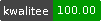++ed by:
1 non-PAUSE user
and 1 contributors

# NAME

LCS::Similar - allow differences in the compared elements of Longest Common Subsequence (LCS) Algorithm# SYNOPSIS

``````  use LCS::Similar;

\$alg = LCS::Similar->new;
@lcs = \$alg->LCS(\@a,\@b);``````

# ABSTRACT

LCS::Similar allows differences in the compared elements.

# DESCRIPTION

Pure LCS algorithms can be used for global alignment of two sequencies.

Usually they provide one result, but there can be more than one solution fulfilling the criterion of being longest.

For example this two sequencies have two possible LCS:

``````   # solution1
@sequence1 = qw/a b   d   /;
@sequence2 = qw/  b a d c /;
@lcs1      = qw/  b   d   /;

# solution2
@sequence1 = qw/  a b d   /;
@sequence2 = qw/b a   d c /;
@lcs2      = qw/  a   d   /;``````

Or an example of mistyping:

``````   eo___nnnnnicaio
|   |    ||| |
commun____icato``````

This is an actual result of a pure LCS algorithm. Here the letter n of the second line could align in 5 possible ways with one of the letters n of the first line, and the LCS will always have a length of 6.

But this solution better maps the mismatches:

``````   eonnnnnicaio
~|~~~ ||||~|
commu_nicato``````

Here the tilde ~ represents similarity of the aligned characters.

With a function returning e.g. a similarity of 0.7 for a comparison of similar characters this module takes similarity into account for alignment.

See examples.

## CONSTRUCTOR

new()

Creates a new object which maintains internal storage areas for the LCS computation. Use one of these per concurrent LCS() call.

## METHODS

LCS(\@a,\@b,\&similarity,\$threshold)

Finds a Longest Common Subsequence, taking two arrayrefs as method arguments. It returns an array reference of corresponding indices, which are represented by 2-element array refs.

The third argument is the reference of a subroutine comparing two elements and returning a number between 0 and 1. Where 0 means unequal and 1 means equal.

Without a subroutine the module falls back to string comparison.

The fourth argument is a threshold passed to the subroutine.

max(\$number1, \$number2)

Returns the maximum of two numbers.

max3(\$number1, \$number2, \$number3)

Returns the maximum of three numbers.

None by design.

# EXAMPLES

## Aline two textfiles

``````  use LCS::Similar;
use LCS;

binmode(STDOUT,":encoding(UTF-8)");

open(my \$in1,"<:encoding(UTF-8)",'file1')
or die "cannot open file1: \$!";
open(my \$in2,"<:encoding(UTF-8)",'file2')
or die "cannot open file2: \$!";

my \$lines1 = [<\$in1>];
my \$lines2 = [<\$in2>];

sub similarity {
my (\$a, \$b, \$threshold) = @_;

\$a //= '';
\$b //= '';
\$threshold //= 0.7;

return 1 if (\$a eq \$b);
return 1 if (!\$a eq !\$b); # avoid division by zero

# length of LCS
my \$llcs = LCS->LLCS(
[split(//,\$a)],
[split(//,\$b)],
);

# the standard formula
my \$similarity = (2 * \$llcs) / (length(\$a) + length(\$b));
return \$similarity if (\$similarity >= \$threshold);
return 0;
}

# aligned indices of elements similar more than \$threshold 0.5
my \$lcs = LCS::Similar->LCS( \$lines1, \$lines2, \&similarity, 0.5 );

# map indices and not so similar elements into AoA of lines
my \$aligned = LCS->lcs2align( \$lines1, \$lines2, \$lcs );

# print them
for my \$chunk (@\$aligned) {
print 'a: ',\$chunk->;
print 'b: ',\$chunk->;
print "\n";
}``````

## Aline two words

``````  use LCS::Similar;
use LCS;

my \$word1 = [ split(//, 'eonnnnnicaio') ];
my \$word1 = [ split(//, 'communicato' ) ];

sub confusable {
my (\$a, \$b, \$threshold) = @_;

\$a //= '';
\$b //= '';
\$threshold //= 0.7;

return 1 if (\$a eq \$b);
return 1 if (!\$a && !\$b);

my \$map = {
'e' => 'c',
'c' => 'e',
'm' => 'n',
'n' => 'm',
'i' => 't',
't' => 'i',
};

return \$threshold if (exists \$map->{\$a} && \$map->{\$a} eq \$b);
return 0;
}

my \$aligned = [
LCS->align2strings(
LCS->lcs2align(
\$word1,
\$word2,
LCS::Similar->LCS( \$word1, \$word2, \&confusable, 0.7 )
)
)
];
print 'a: ',\$aligned->,"\n"; # eonnnnnicaio
print 'b: ',\$aligned->,"\n"; # commu_nicato
print "\n";``````

Algorithm::Diff

# AUTHOR

Helmut Wollmersdorfer <helmut.wollmersdorfer@gmail.com>